Home > CCA2 > Chapter 8 > Lesson 8.2.2 > Problem8-87

8-87.
1. For each of the following sets of numbers, find the equation of a function that has these numbers as roots. Homework Help ✎

1. −3 + i and −3 − i

2. 5 +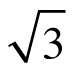and 5 −3. −2,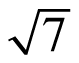, and −4. 4, −3 + i, and −3 − i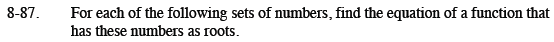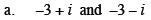If these are the roots, what are the factors?

$y=\left(x-(-3+i)\right)\left(x-(-3-i)\right)$

$y=(x+3-i)(x+3+i)$

Multiply the factors.
You may want to use a generic rectangle.
The first row has been filled in for you.
Simplify after multiplying.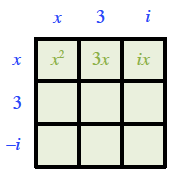$f(x)=x^2+6x +10$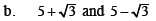Use the same process as part (a).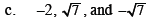What are the factors?

$y=(x-(-2))(x-\sqrt{7})(x+\sqrt{7})$

Multiply the last two factors first.
Then multiply the result by (x + 2).

$h(x)=x^3+2x^2-7x-14$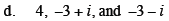Use the ideas from part (a) and (c) to help you.
How can you use the results from part (a) to make this problem easier?